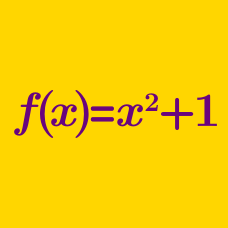Algebra

# Functions: Level 5 Challenges

Let $f(x)$ be a cubic polynomial such that $f(1) = 5, f(2) = 20, f(3) = 45$.

Then find the product of roots of the equation below.

$\large [f(x)]^{2} + 3x \ f(x) + 2x^{2} = 0$

Let $f$ be a function from the integers to the real numbers such that $f(x) = f(x-1) \cdot f(x+1).$

What is the maximum number of distinct values of $f(x)$?

Let $f(x)$ be a polynomial. It is known that for all $x$,

$\large f(x)f(2x^2) = f(2x^3+x)$

If $f(0)=1$ and $f(2)+f(3)=125$, find $f(5)$.

Given a function $f$ for which $f(x) = f(398 - x) = f(2158 - x) = f(3214 - x)$ holds for all real $x$, what is the largest number of different values that can appear in the list $f(0),f(1),f(2),\ldots,f(999)?$

The functions $f(x)$ and $g(x)$ are defined $\mathbb {R^+ \to R}$ such that $f(x)=\begin{cases} 1-\sqrt{x}\quad \text{x is rational} \\ \quad x^2\quad\quad\text{x is irrational}\end{cases}\\g(x)=\begin{cases} \quad x\quad\quad~~~ \text{x is rational} \\ 1-x\quad\quad\text{x is irrational}\end{cases}$

The composite function $f \circ g(x)$ is

×